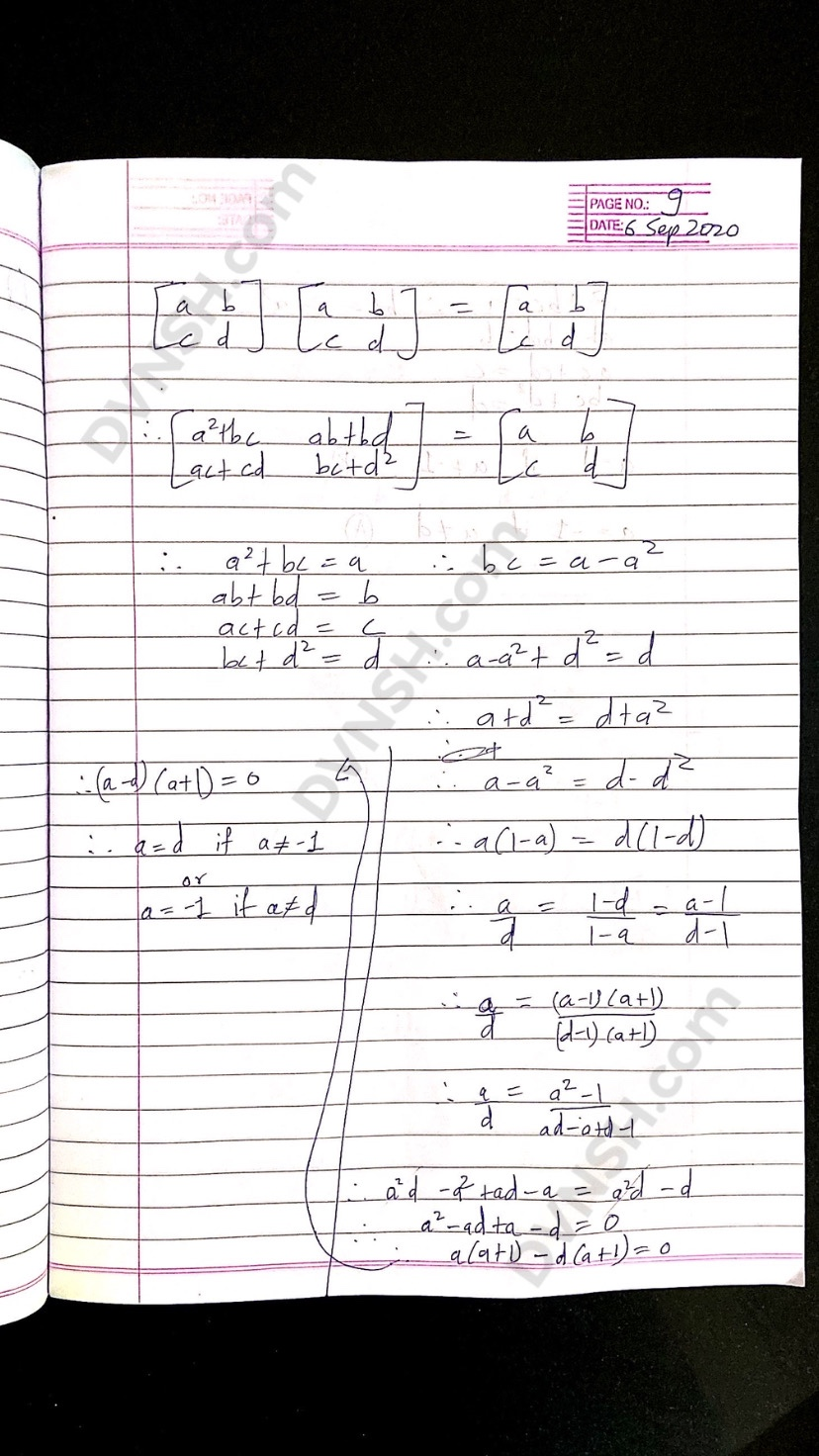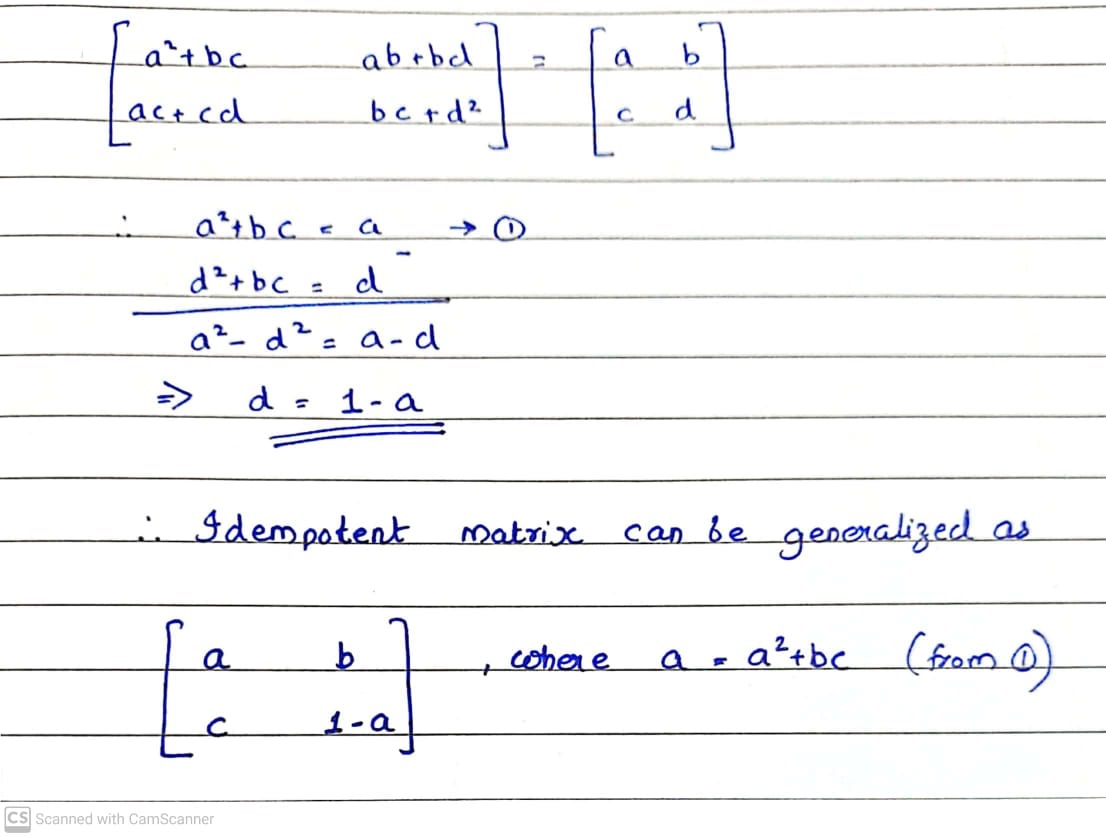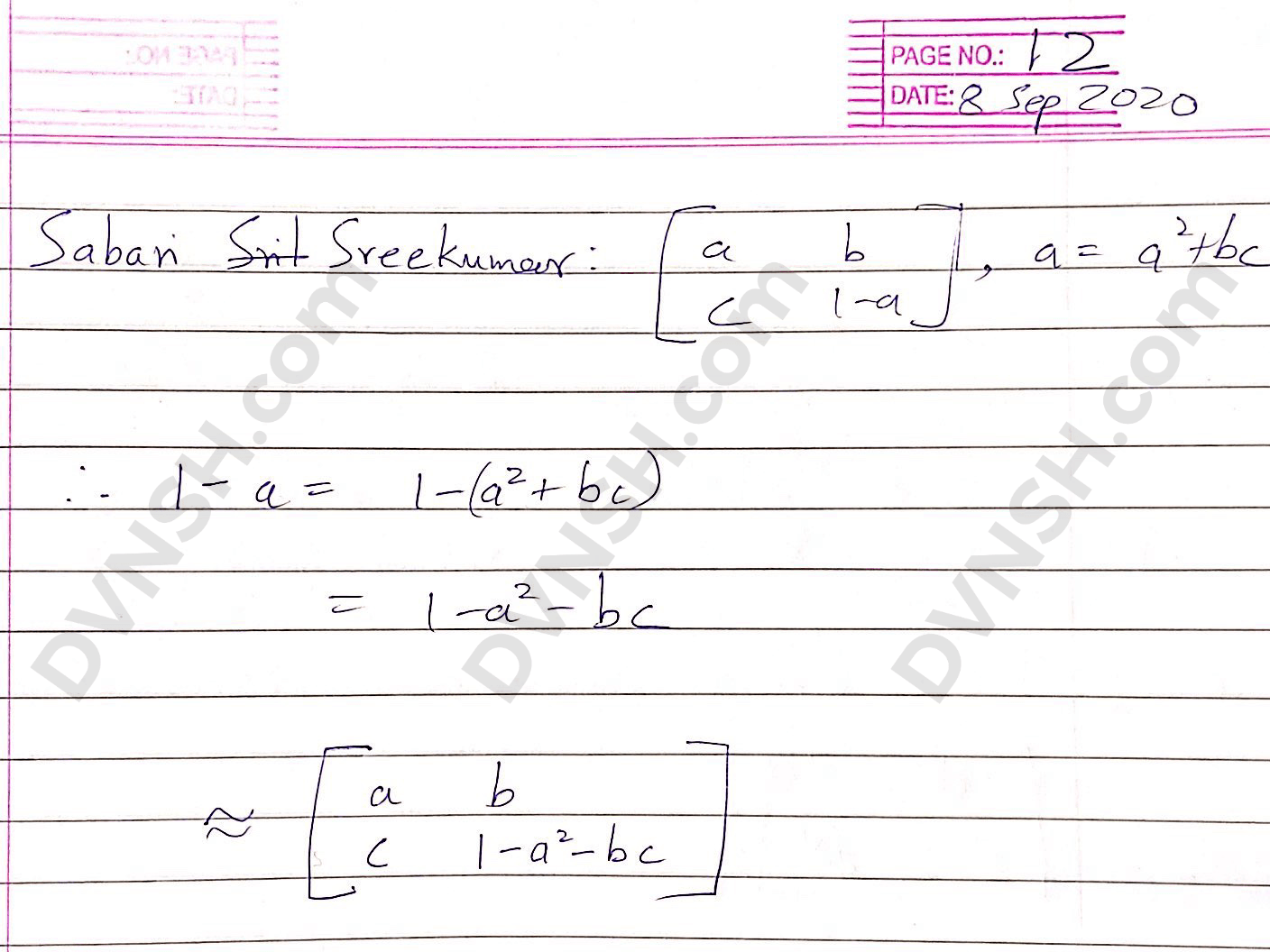# 2 X 2 Idempotent matrix

This post is a part of my elective course Mathematical Foundations for Data Science during my M. Tech. in Software Systems with specialization in Data Analytics.

I had to provide an example of an idempotent matrix. That’s the kind of matrix that yields itself when multiplied to itself. Much like 0 and 1 in scalar multiplication (1 x 1 = 1).

It is not so easy to predict the result of a matrix multiplication, especially for large matrices. So, instead of settling with the naïve method of guessing with trial and error, I explored the properties of a square matrix of the order 2.In this page I state the question and begin to attempt it. I realised that for a matrix to be idempotent, it would have to retain its dimensions (order), and hence be a square matrix.I have intentionally put distinct variable names a,b,c, and d. This is to ensure that the possibility of a different number at each index is open. I derived ‘bc’ from the first equation and substituted it into its instance in the last equation to obtain a solution for ‘a’.

Since 0 cannot be divided by 0, I could not divide 0 by either term unless it was a non-zero term. Thus, I had two possibilities, to which I called case A and B.I solved the four equations in case A by making substitutions into the 4 main equations. Later tested the solution with b=1.

As you can see, I could not use the elimination method in an advantageous manner for this case.

I couldn’t get a unique solution in either case. That is because there are many possible square matrices that are idempotent. However, I don’t feel comfortable to intuit that every 2 X 2 idempotent matrix has one of only two possible numbers as its first and last elements.

## Others’ take on itMy classmate Sabari Sreekumar did manage to use elimination for the ‘bc’ term for the general case.I took it a step further and defined the last element in terms of the other elements

So given any 2 X 2 idempotent matrix and its first three elements, you can find the last element unequivocally with this formula.

## Conclusion

I wonder if multiples of matrices that satisfy either case are also idempotent. Perhaps I will see if I can prove that in another post.

In the next lecture, professor Venkata Ratnam suggested using the sure-shot approach of a zero matrix. And I was like “Why didn’t I think of that”?